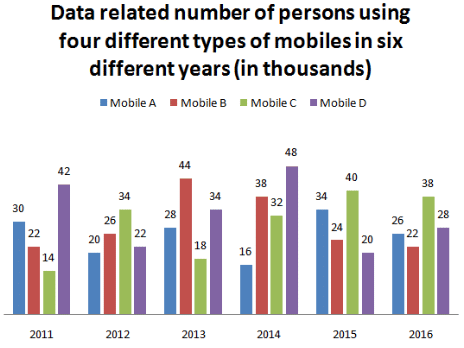Direction: Read the following graph carefully and answer the question given below.What is the sum of the number of persons using mobile A and C together in 2015 and number of persons using mobile B and D in 2016?
• a)
124250
• b)
124200
• c)
124100
• d)
124500
• e)
124000

### Related TestConstructing Careers Feb 11, 2022Number of persons using mobile A and C together in 2015
= (34 + 40) × 1000 = 74000
Number of persons using mobile B and D together in 2016
=(22 + 28) × 1000 = 50000
Sum = 74000 + 50000 = 124000
Hence, the correct option is (E).

Number of persons using mobile A and C together in 2015= (34 + 40) × 1000 = 74000Number of persons using mobile B and D together in 2016=(22 + 28) × 1000 = 50000Sum = 74000 + 50000 = 124000Hence, the correct option is (E).
Do you know? How Toppers prepare for CAT Exam
With help of the best CAT teachers & toppers, We have prepared a guide for student who are preparing for CAT : 15 Steps to clear CAT Exam